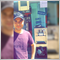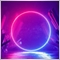# Error code : '&&' - program is too complex163

Hello

(At MQL5)

When i try to input 5000 conditions in my code it gives me this error :

'&&' - program is too complex

After i remove some conditions then the code work perfectly

How can i fix this error without removing any conditions?

is mq5 is a limited conditions ?26008

1. If you have 5K conditions, the problem is your brain. Do not cut and paste identical conditions. Show us an example if you want help.
The XY Problem

2. Simplify and combine.
```bool c0010 = condition01 && condition02 … && condition09;
bool c0020 = …
⋮
bool C0100 = c0010 && c0020 … && c0090;
bool C0200 = …
⋮
bool c5000 = c1000 && c2000 …```163

William Roeder #:
1. If you have 5K conditions, the problem is your brain. Do not cut and paste identical conditions. Show us an example if you want help.
The XY Problem

2. Simplify and combine.

This is my code:

```//--- indicator settings
#property indicator_chart_window
#property indicator_buffers 2
#property indicator_plots 2

#property indicator_type1 DRAW_ARROW
#property indicator_width1 3
#property indicator_color1 0xFFAA00

#property indicator_type2 DRAW_ARROW
#property indicator_width2 3
#property indicator_color2 0xEA00FF
#property indicator_label2 "Sell"

//--- indicator buffers
double Buffer1[];
double Buffer2[];

double myPoint; //initialized in OnInit
double Open[];
double Close[];
int RSI_handle;
double RSI[];
double Low[];
double High[];

{
if(type == "print")
Print(message);
else if(type == "error")
{
Print(type+" | ZoozFX @ "+Symbol()+","+IntegerToString(Period())+" | "+message);
}
else if(type == "order")
{
}
else if(type == "modify")
{
}
}

//+------------------------------------------------------------------+
//| Custom indicator initialization function                         |
//+------------------------------------------------------------------+
int OnInit()
{
SetIndexBuffer(0, Buffer1);
PlotIndexSetDouble(0, PLOT_EMPTY_VALUE, EMPTY_VALUE);
PlotIndexSetInteger(0, PLOT_ARROW, 241);
SetIndexBuffer(1, Buffer2);
PlotIndexSetDouble(1, PLOT_EMPTY_VALUE, EMPTY_VALUE);
PlotIndexSetInteger(1, PLOT_ARROW, 242);
//initialize myPoint
myPoint = Point();
if(Digits() == 5 || Digits() == 3)
{
myPoint *= 10;
}
RSI_handle = iRSI(NULL, PERIOD_CURRENT, 11, PRICE_CLOSE);
if(RSI_handle < 0)
{
Print("The creation of iRSI has failed: RSI_handle=", INVALID_HANDLE);
Print("Runtime error = ", GetLastError());
return(INIT_FAILED);
}

return(INIT_SUCCEEDED);
}

//+------------------------------------------------------------------+
//| Custom indicator iteration function                              |
//+------------------------------------------------------------------+
int OnCalculate(const int rates_total,
const int prev_calculated,
const datetime& time[],
const double& open[],
const double& high[],
const double& low[],
const double& close[],
const long& tick_volume[],
const long& volume[],
{
int limit = rates_total - prev_calculated;
//--- counting from 0 to rates_total
ArraySetAsSeries(Buffer1, true);
ArraySetAsSeries(Buffer2, true);
//--- initial zero
if(prev_calculated < 1)
{
ArrayInitialize(Buffer1, EMPTY_VALUE);
ArrayInitialize(Buffer2, EMPTY_VALUE);
}
else
limit++;

if(CopyOpen(Symbol(), PERIOD_CURRENT, 0, rates_total, Open) <= 0) return(rates_total);
ArraySetAsSeries(Open, true);
if(CopyClose(Symbol(), PERIOD_CURRENT, 0, rates_total, Close) <= 0) return(rates_total);
ArraySetAsSeries(Close, true);
if(BarsCalculated(RSI_handle) <= 0)
return(0);
if(CopyBuffer(RSI_handle, 0, 0, rates_total, RSI) <= 0) return(rates_total);
ArraySetAsSeries(RSI, true);
if(CopyLow(Symbol(), PERIOD_CURRENT, 0, rates_total, Low) <= 0) return(rates_total);
ArraySetAsSeries(Low, true);
if(CopyHigh(Symbol(), PERIOD_CURRENT, 0, rates_total, High) <= 0) return(rates_total);
ArraySetAsSeries(High, true);
//--- main loop
for(int i = limit-1; i >= 0; i--)
{
if (i >= MathMin(450000-1, rates_total-1-5100)) continue; //omit some old rates to prevent "Array out of range" or slow calculation

//Indicator Buffer 1

if((Close[i] > Open[i]&& Close[1+i] > Open[1+i]&& Low[i] > High[2+i]&& RSI[2+i] < 30)
|| (Close[1+i] > Open[1+i]&& Close[2+i] > Open[2+i]&& Low[1+i] > High[3+i]&& RSI[3+i] < 30)
)
{
Buffer1[i] = Low[i]; //Set indicator value at Candlestick Low
}
else
{
Buffer1[i] = EMPTY_VALUE;
}
//Indicator Buffer 2

if((Close[i] > Open[i]&& Close[1+i] > Open[1+i]&& Low[i] > High[2+i]&& RSI[2+i] < 30)
|| (Close[1+i] > Open[1+i]&& Close[2+i] > Open[2+i]&& Low[1+i] > High[3+i]&& RSI[3+i] < 30)
)
{
Buffer2[i] = High[i]; //Set indicator value at Candlestick High
}
else
{
Buffer2[i] = EMPTY_VALUE;
}
}
return(rates_total);
}
//+------------------------------------------------------------------+```

I want to repeat the next condition 5000 times every time change it to become like this

`  || (Close[1+i] > Open[1+i]&& Close[2+i] > Open[2+i]&& Low[1+i] > High[3+i]&& RSI[3+i] < 30)`
`|| (Close[2+i] > Open[2+i]&& Close[3+i] > Open[3+i]&& Low[2+i] > High[4+i]&& RSI[4+i] < 30)`

`|| (Close[3+i] > Open[3+i]&& Close[4+i] > Open[4+i]&& Low[3+i] > High[5+i]&& RSI[5+i] < 30)`

After i do this 5000 times its gives me that error

How can i use this "bool c5000" in this case?163

??5055

Ahmed Abd El Aziz #:

This is my code:

I want to repeat the next condition 5000 times every time change it to become like this

After i do this 5000 times its gives me that error

How can i use this "bool c5000" in this case?

Just use a loop:

```bool conditionMet = false;
for (int j = 0; j < 5000; j++) {
if (Close[j+i] > Open[j+i] && Close[j+i] > Open[j+i]&& Low[j+i] > High[j+i]&& RSI[j+i] < 30) {
conditionMet = true;
// Break out of the loop.
break;
}
}

if (conditionMet) {
Buffer1[i] = Low[i]; //Set indicator value at Candlestick Low
} else {
Buffer1[i] = EMPTY_VALUE;
}```163

Alexandre Borela #:

Just use a loop:

You are the best

I did it. Thank you very much